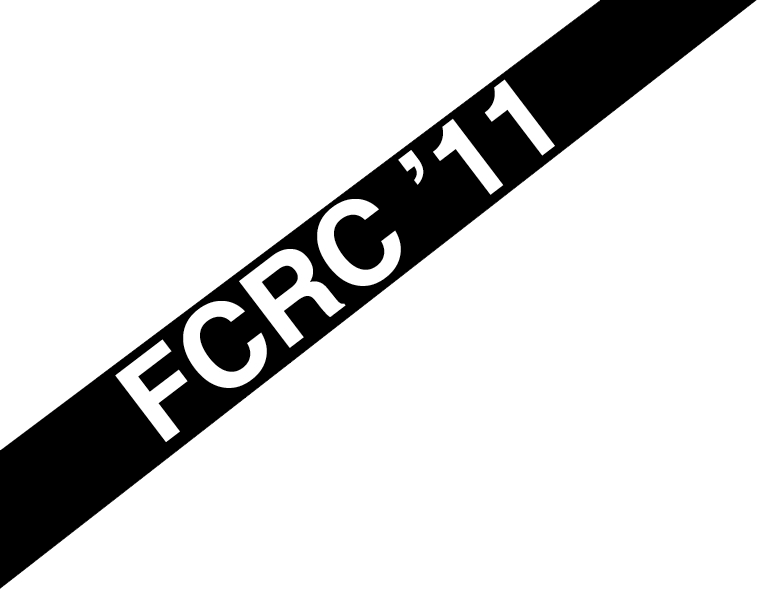# Invited Talks

Victor S. Miller and Gilles Villard are the two invited speakers for ISSAC 2011.

 Victor S. Miller Center for Communications Research, Princeton, USA

## Computational Aspects of Elliptic Curves and Modular Forms

One of the ultimate driving forces behind Number Theory is the solution of Diophantine Equations: solutions of polynomials systems in integers or rational numbers. Perhaps the most infamous is “Fermat’s Last Theorem” (solved by Andrew Wiles) xn + yn = zn. For n = 3 (a case treated by Fermat) this is an instance of an “elliptic curve”. Elliptic Curves are the first really hard class of diophantine equations, and have had a huge impact on all of number theory. The study of elliptic curves has always been interwoven with large and difficult calculations — the results of which have spurred the theory (and vice versa). The famous Birch and Swinnerton-Dyer conjecture (which is one of Clay Mathematics Institute \$1 million problems) was only formuated after extensive computer computations, which we will describe. The “Modularity Conjecture” (aka the Shimura-Taniyama-Weil conjecture) was also buttressed by extensive computations with elliptic curves and modular forms. It was this conjecture that Wiles proved. It had been previously proved by Frey and Ribet that this conjecture implied Fermat’s Last Theorem.

Elliptic Curves have also found applications in cryptography — by means of the presumed difficulty of the ECDLP (Elliptic Curve discrete logarithm problem), and in the new and burgeoning field of pairing based cryptography.

In this lecture we will discuss some of the important algorithms connected with Elliptic Curves, and illustrate some of the computational data which lead to the formulation of the Birch Swinnerton-Dyer conjecture.

 Gilles Villard, CNRS-Université de Lyon, France

## Recent progress in linear algebra for lattice basis reduction

We describe some recent progress in lattice basis reduction algorithms. For univariate polynomial matrices the question consists in computing a column reduced form. The form is a useful tool for example in linear control or computer algebra. It has received a lot of attention, a main target being to lower the cost to essentially the same as that of multiplying two polynomial matrices. For integer Euclidean lattice bases we are interested in the algorithmics of the LLL reduction. The LLL reduction is a cornerstone of many lattice applications in computer science and computational mathematics. Despite being polynomial-time, the best reduction algorithms remain somewhat slow and there might be room for improvement.

Using a linear algebra point of view we will present some analogies between recent algorithms in the polynomial and the integer cases that rely on minimal approximant basis computations or gradual feeding. Main ingredients involved are matrix normal forms and sensitivity results on matrix factorizations. We will also see that the notion of shifted reduction allows to adapt the Lehmer-Knuth-Schönhage framework to both the polynomial and integer cases.

References.

A. K. Lenstra, H. W. Lenstra, Jr., and L. Lovász. Factoring polynomials with rational coefficients. Mathematische Annalen, 261:515–534, 1982.

B. Beckermann and G. Labahn. A uniform approach for the fast computation of matrix-type Padé approximants. SIAM Journal on Matrix Analysis and Applications, 15(3):804–823, 1994.

P. Giorgi, C.P. Jeannerod and G. Villard. On the complexity of polynomial matrix computations. In Proc. International Symposium on Symbolic and Algebraic Computation, Philadelphia, Pennsylvania USA, aug. 2003, 135–142, ACM Press.

M. van Hoeij and A. Novocin. Gradual sub-lattice reduction and a new complexity for factoring polynomials. In Proc. 9th Latin American Theoretical Informatics Symposium LATIN 2010, LNCS 6034, 539–553. Springer-Verlag, 2010.

A. Novocin, D. Stehlé, G. Villard. An LLL-Reduction algorithm with quasi-linear time complexity. In Proc. 43rd ACM Symposium on Theory of Computing, June 6–8, 2011, San Jose, CA USA.